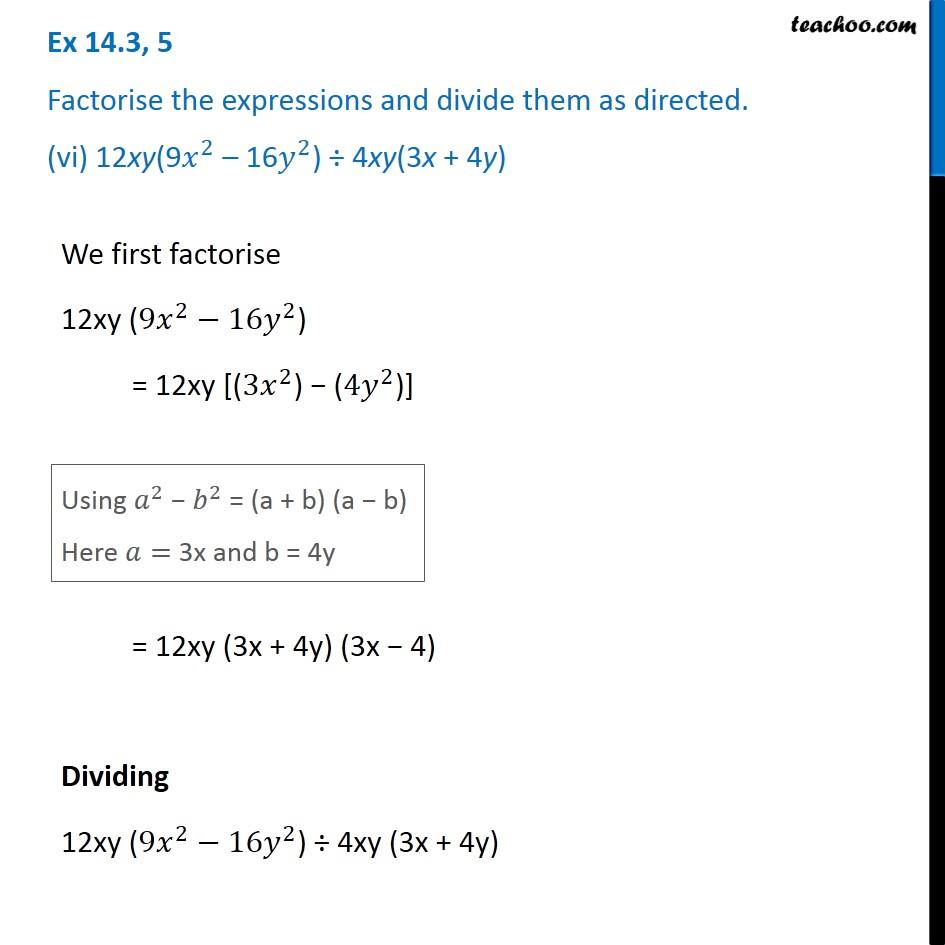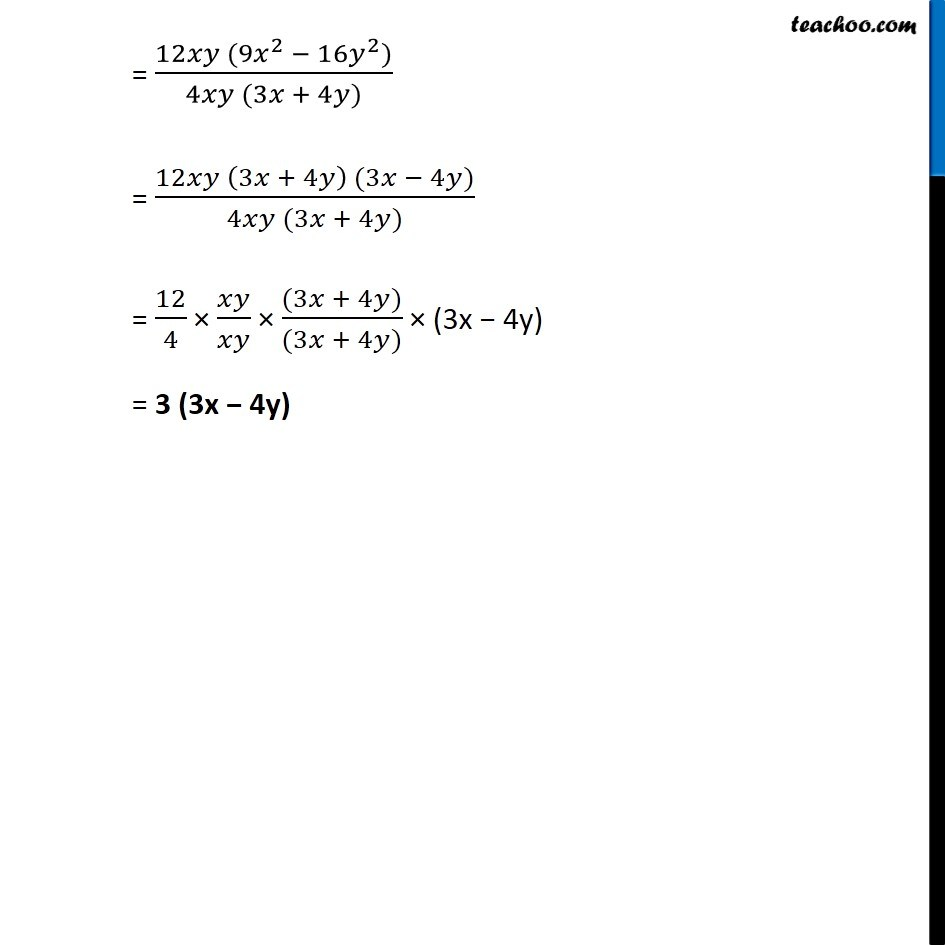Ex 14.3

Chapter 14 Class 8 Factorisation
Serial order wiseGet live Maths 1-on-1 Classs - Class 6 to 12

### Transcript

Ex 14.3, 5 Factorise the expressions and divide them as directed. (vi) 12xy(9𝑥^2 – 16𝑦^2) ÷ 4xy(3x + 4y) We first factorise 12xy (〖9𝑥〗^2−16𝑦^2) = 12xy [(〖3𝑥〗^2) − (〖4𝑦〗^2)] We first factorise 12xy (〖9𝑥〗^2−16𝑦^2) = 12xy [(〖3𝑥〗^2) − (〖4𝑦〗^2)] Using 𝑎^2 − 𝑏^2 = (a + b) (a − b) Here 𝑎= 3x and b = 4y Using 𝑎^2 − 𝑏^2 = (a + b) (a − b) Here 𝑎= 3x and b = 4y = (12𝑥𝑦 (9𝑥^2 − 16𝑦^2))/(4𝑥𝑦 (3𝑥 + 4𝑦)) = (12𝑥𝑦 (3𝑥 + 4𝑦) (3𝑥 − 4𝑦))/(4𝑥𝑦 (3𝑥 + 4𝑦)) = 12/4 × 𝑥𝑦/𝑥𝑦 × ((3𝑥 + 4𝑦))/((3𝑥 + 4𝑦)) × (3x − 4y) = 3 (3x − 4y)